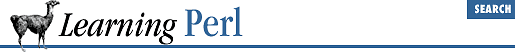home | O'Reilly's CD bookshelfs | FreeBSD | Linux | Cisco | Cisco Exam

### 9.5 Expression Modifiers

As Yet Another Way to indicate "if this, then that," Perl allows you to tag an if modifier onto an expression that is a standalone statement, like this:

``````

some_expression
```
if ```

control_expression
```
;```

In this case, ``` control_expression ``` is evaluated first for its truth value (using the same rules as always), and if true, ``` some_expression ``` is evaluated next. This is roughly equivalent to

```if (```

control_expression
```
) {
```

some_expression
```
;
}```

except that you don't need the extra punctuation, the statement reads backwards, and the expression must be a simple expression (not a block of statements). Many times, however, this inverted description turns out to be the most natural way to state the problem. For example, here's how you can exit from a loop when a certain condition arises:

```LINE: while (<STDIN>) {

last LINE if /^From: /;
}```

See how much easier that is to write? And you can even read it in a normal English way: "last line if it begins with From."

Other parallel forms include the following:

``````

exp2
```

unless ```

exp1
```
; # like: unless (```

exp1
```
) { ```

exp2
```
; }
```

exp2
```

while ```

exp1
```
;  # like: while (```

exp1
```
) { ```

exp2
```
; }
```

exp2
```

until ```

exp1
```
;  # like: until (```

exp1
```
) { ```

exp2
```
; }```

All of these forms evaluate ``` exp1 ``` first, and based on that, do or don't do something with ``` exp2 ``` .

For example, here's how to find the first power of two greater than a given number:

```chomp(\$n = <STDIN>);
\$i = 1;                 # initial guess
\$i *= 2 until \$i > \$n;  # iterate until we find it```

Once again, we gain some clarity and reduce the clutter.

These forms don't nest: you can't say ``` exp3 ``` ``` while``` ``` exp2 ``` ``` if``` ``` exp1 ``` . This is because the form ``` exp2 ``` ``` if``` ``` exp1 ``` is no longer an expression, but a full-blown statement, and you can't tack one of these modifiers on after a statement.9.4 Labeled Blocks9.6 && and || as Control Structures